# 台大李宏毅Machine Learning 2017Fall学习笔记 (6)Logistic Regression

Step 1: Function Set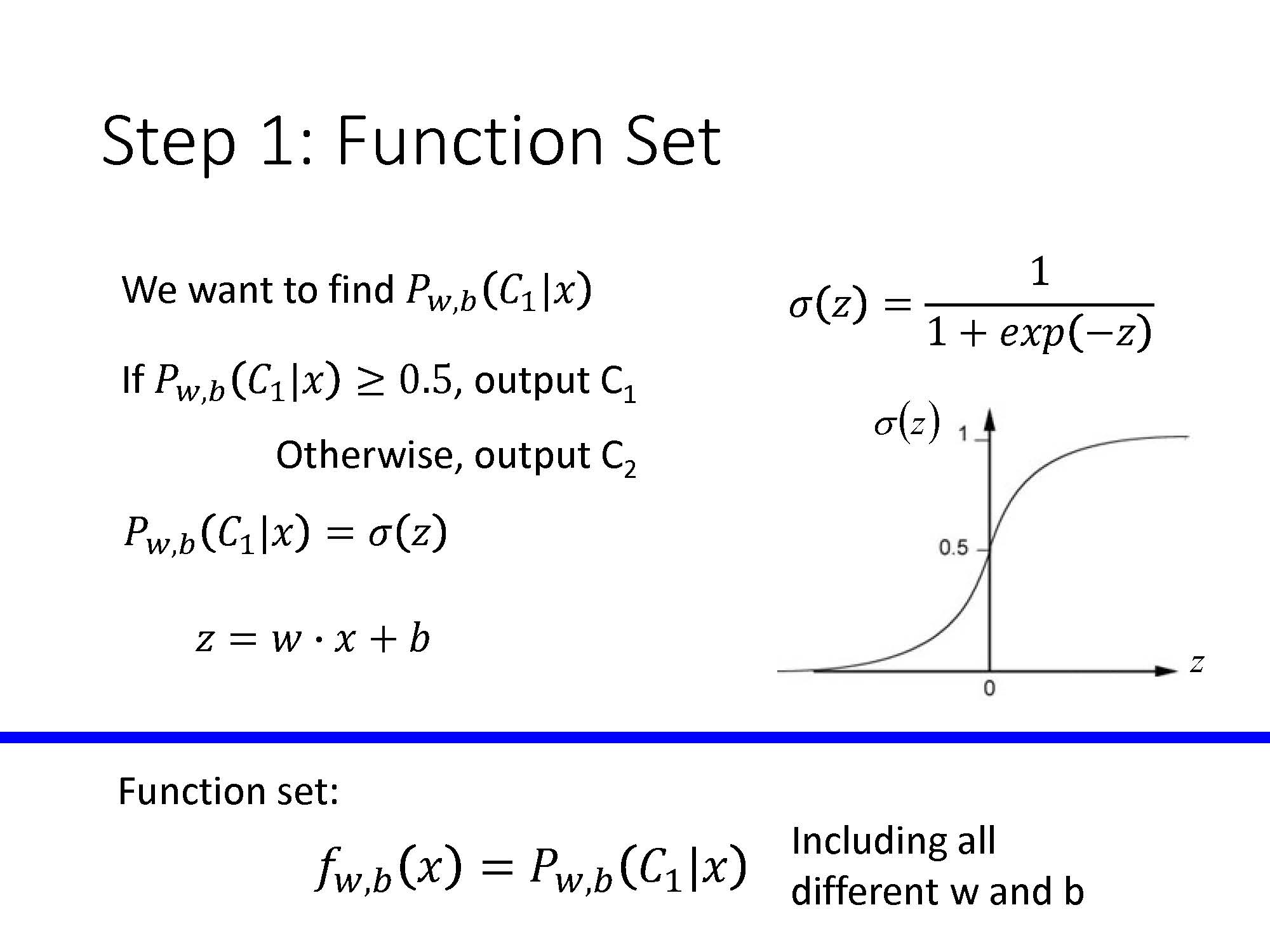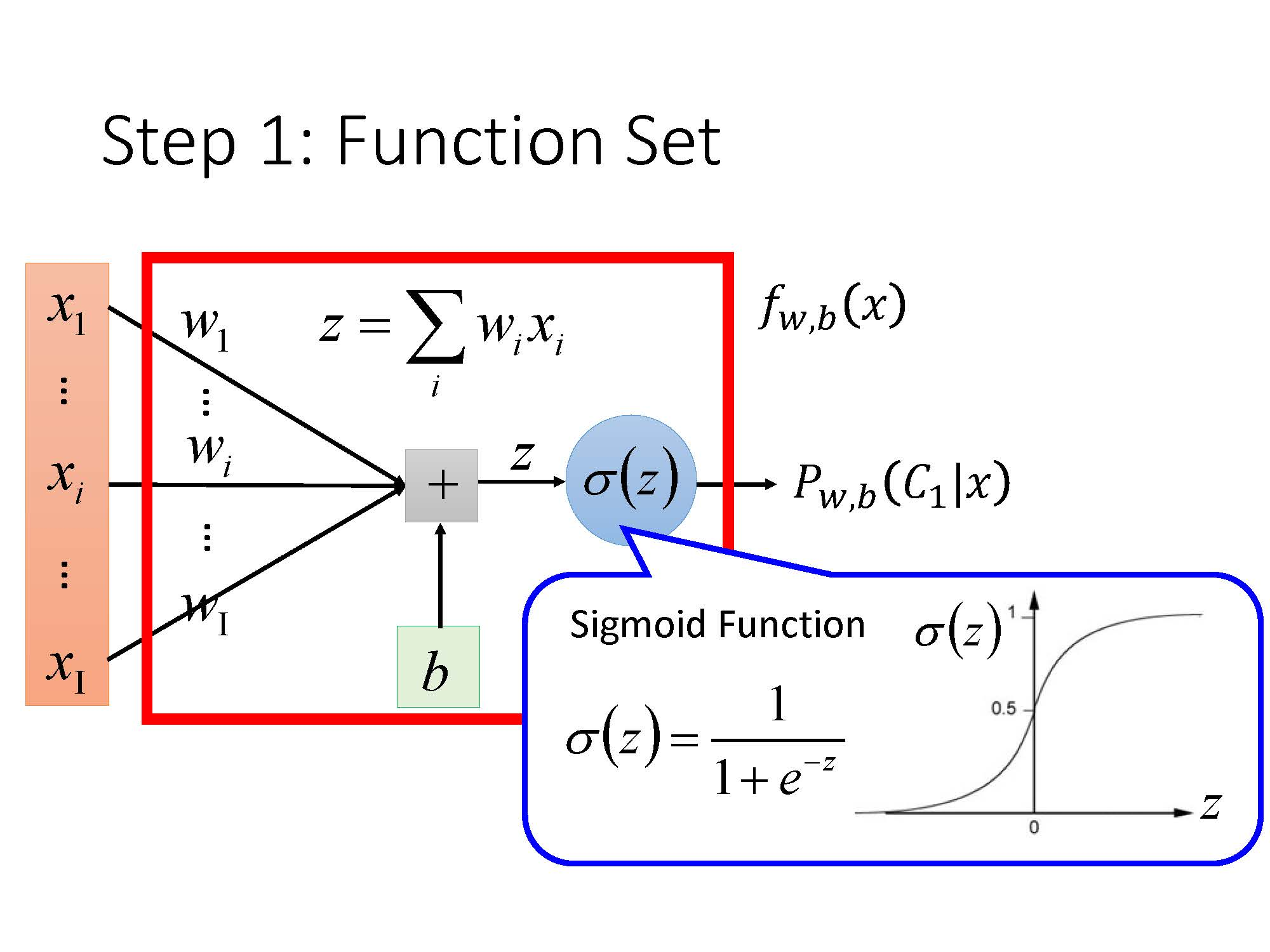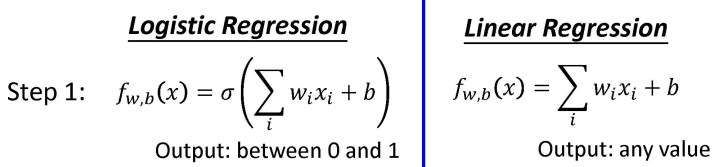Step 2: Goodness of a Function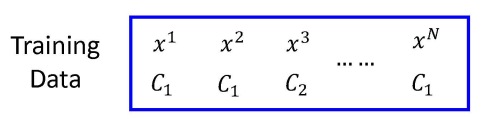L(w,b)=fw,b(x1)fw,b(x2)(1fw,b(x3))fw,b(xN)

w,b=arg maxw,bL(w,b)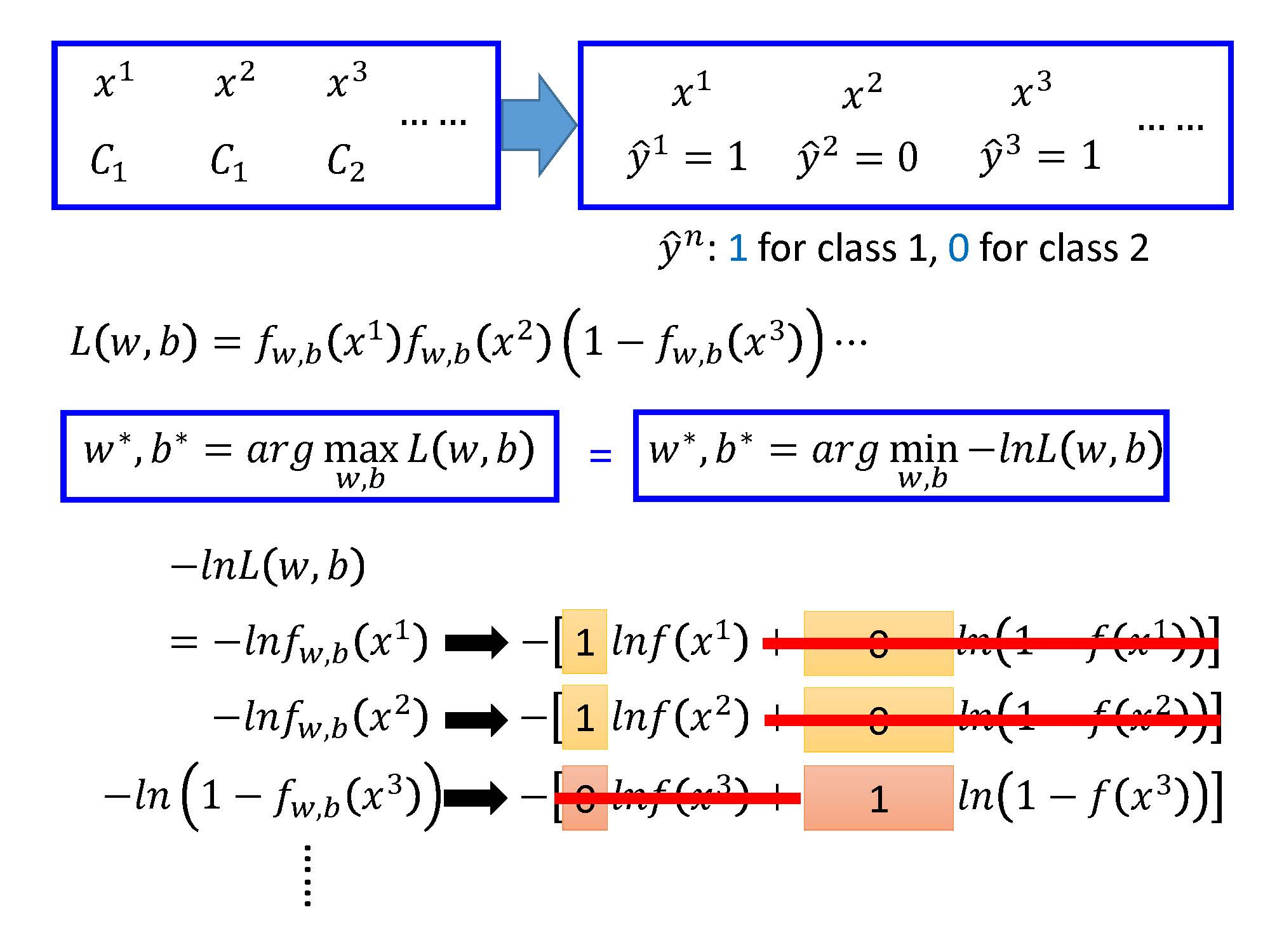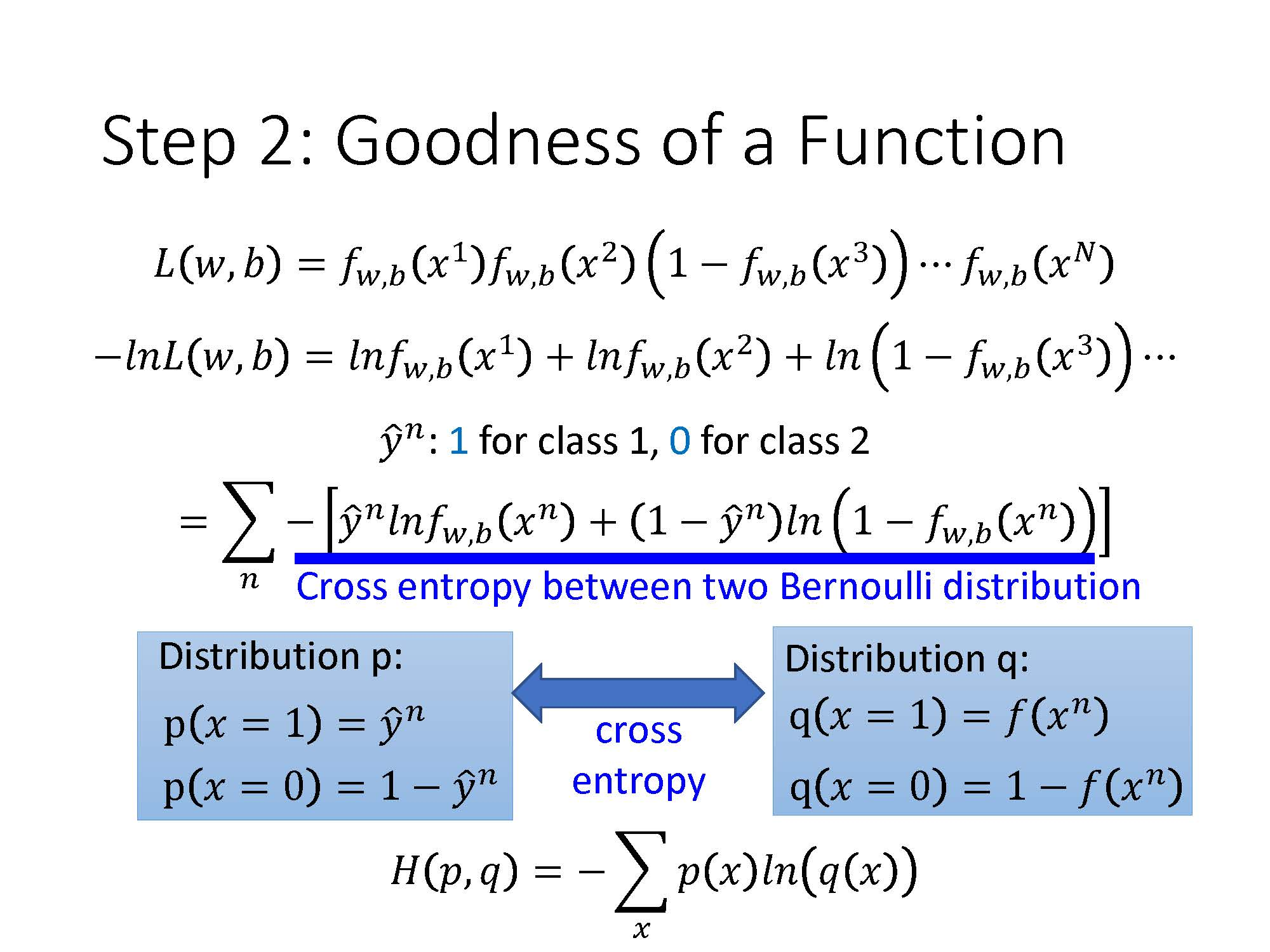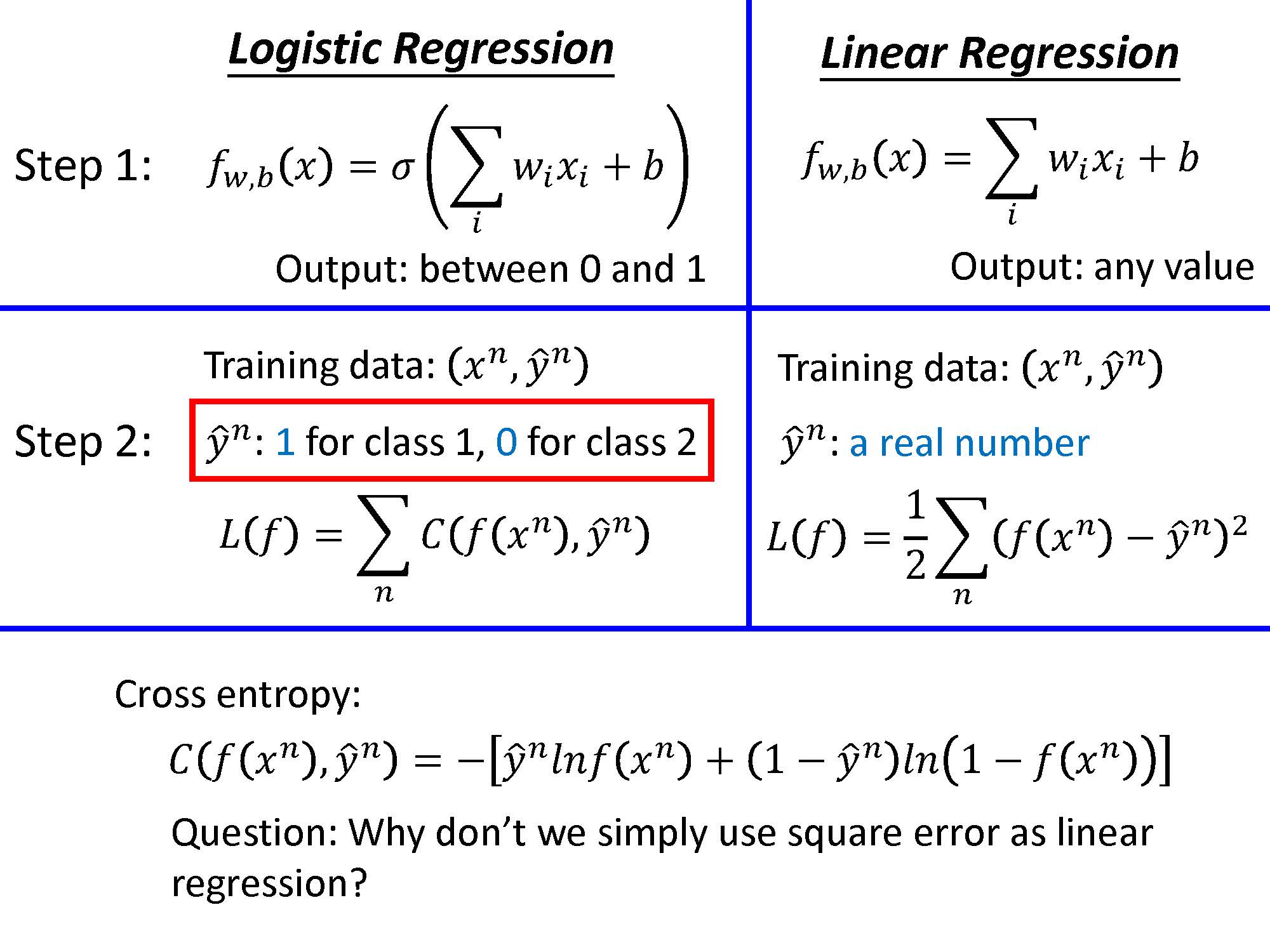Step 3: Find the best function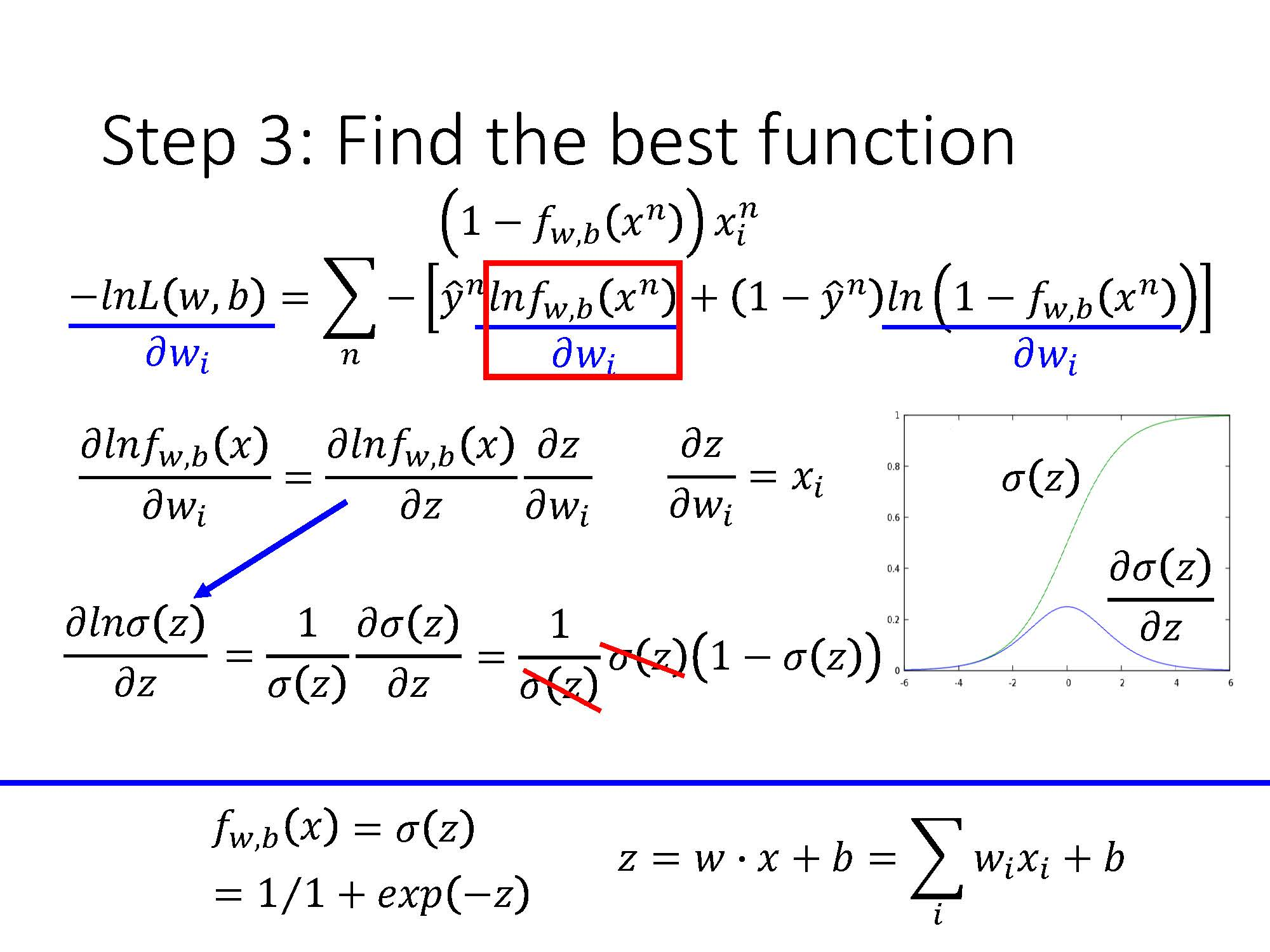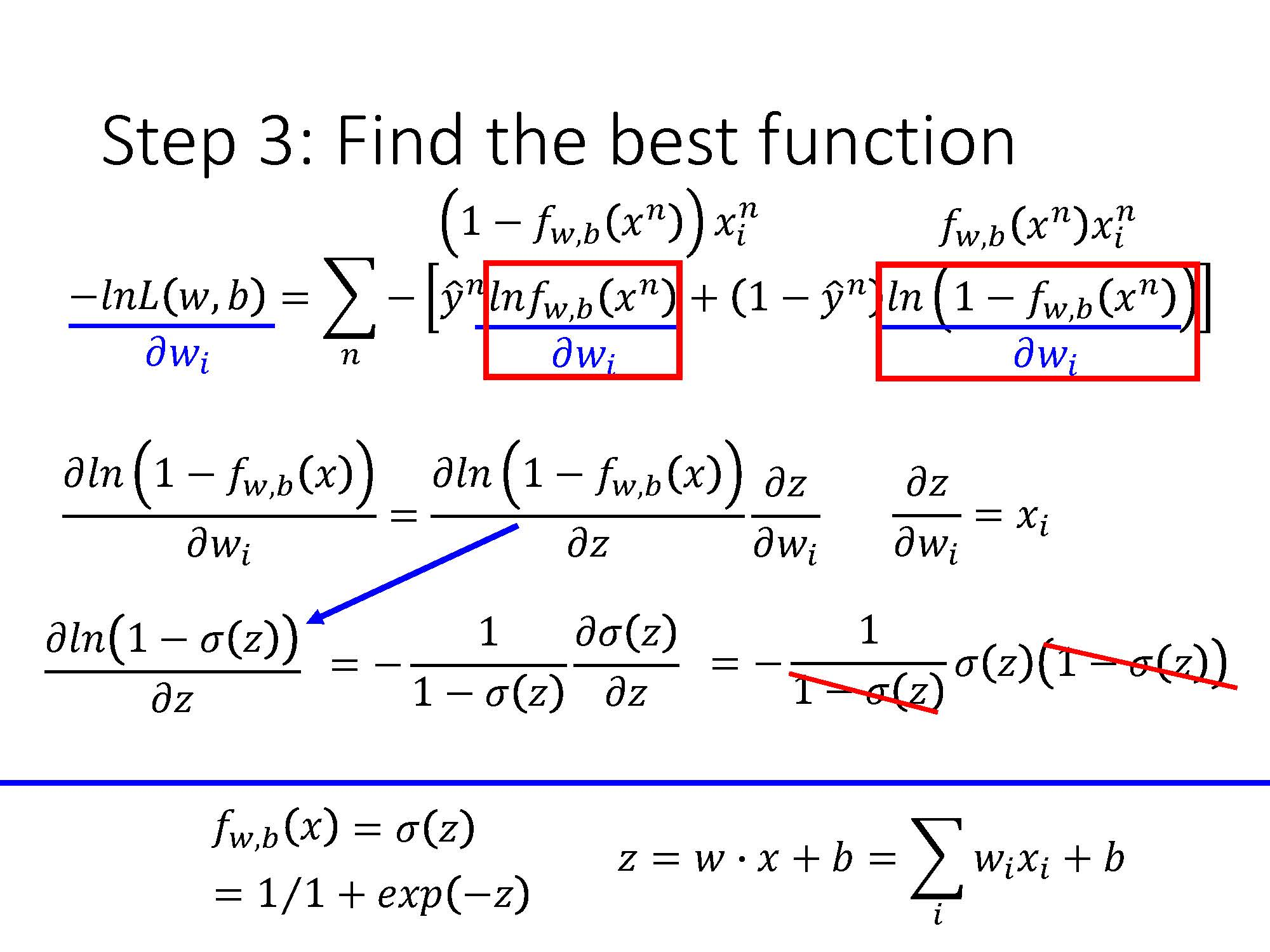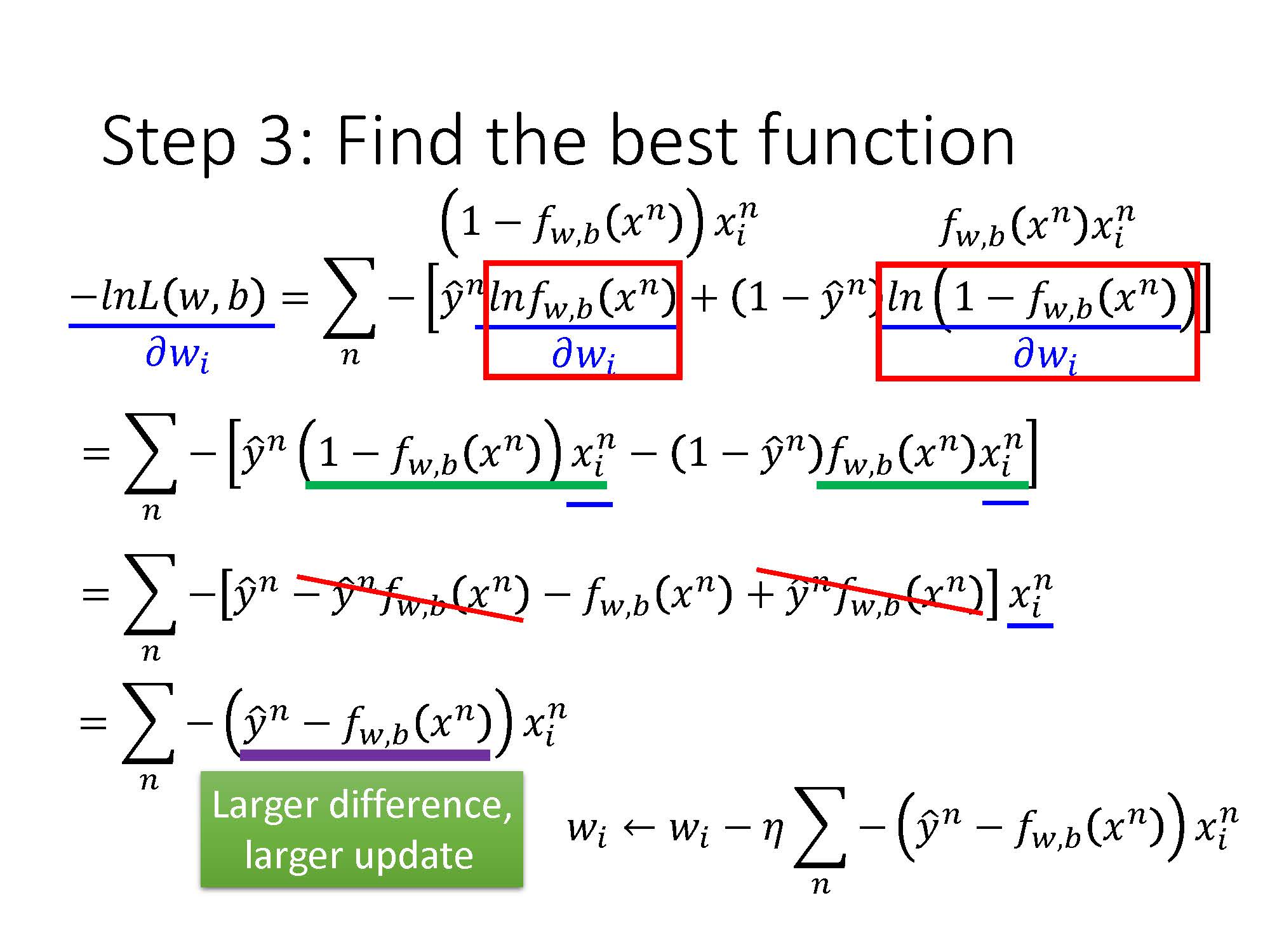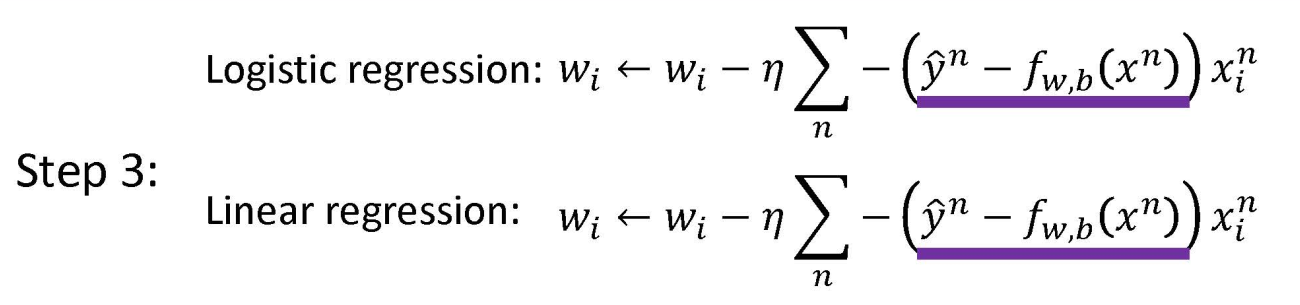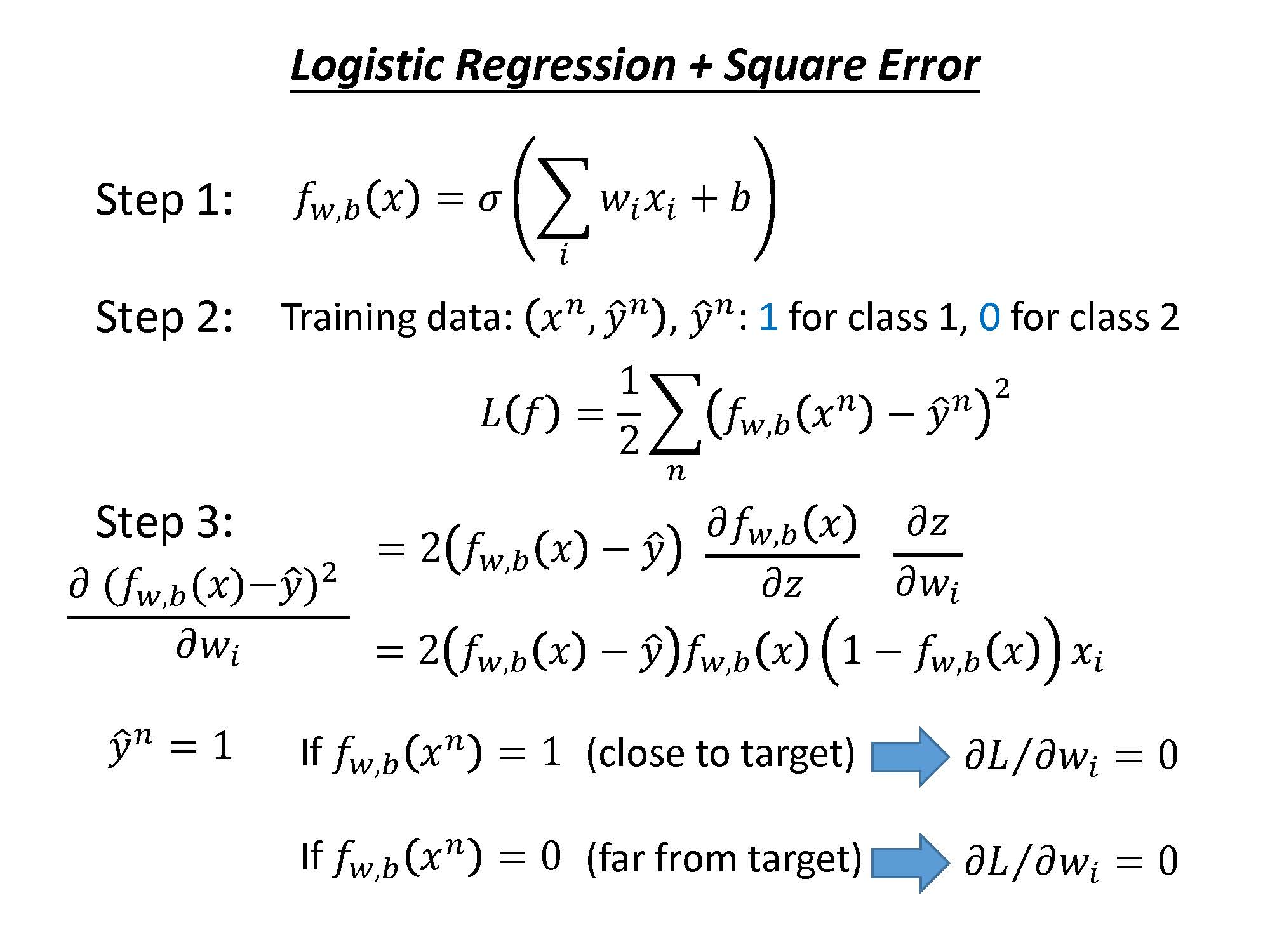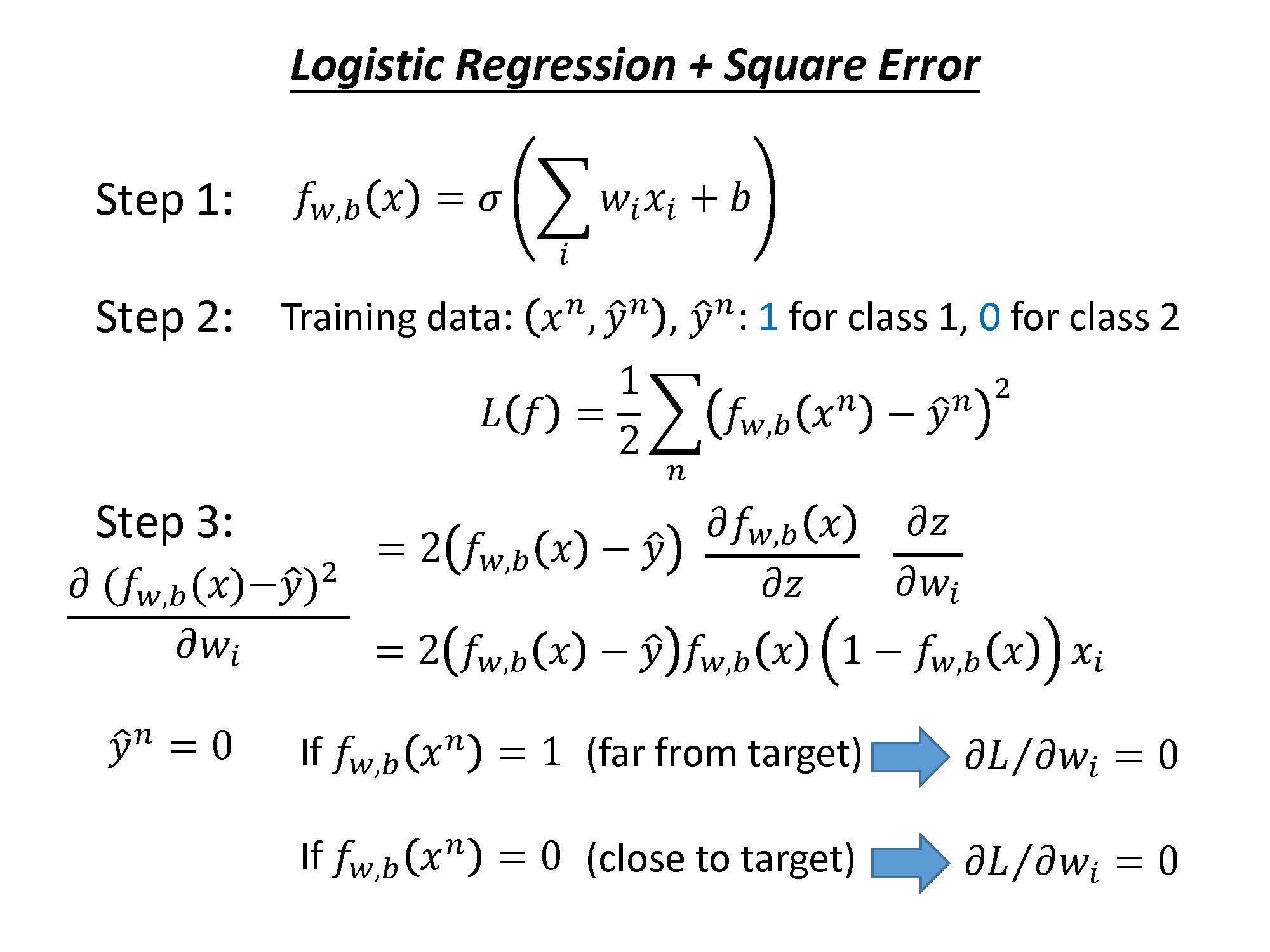Cross entropy$Cross\ entropy$: 距离目标点越远时，目标值越大，参数更新的越快。
Square error$Square\ error$: 当距离目标远时，目标函数对参数的微分值极小，参数更新速度很慢，不容易得到结果。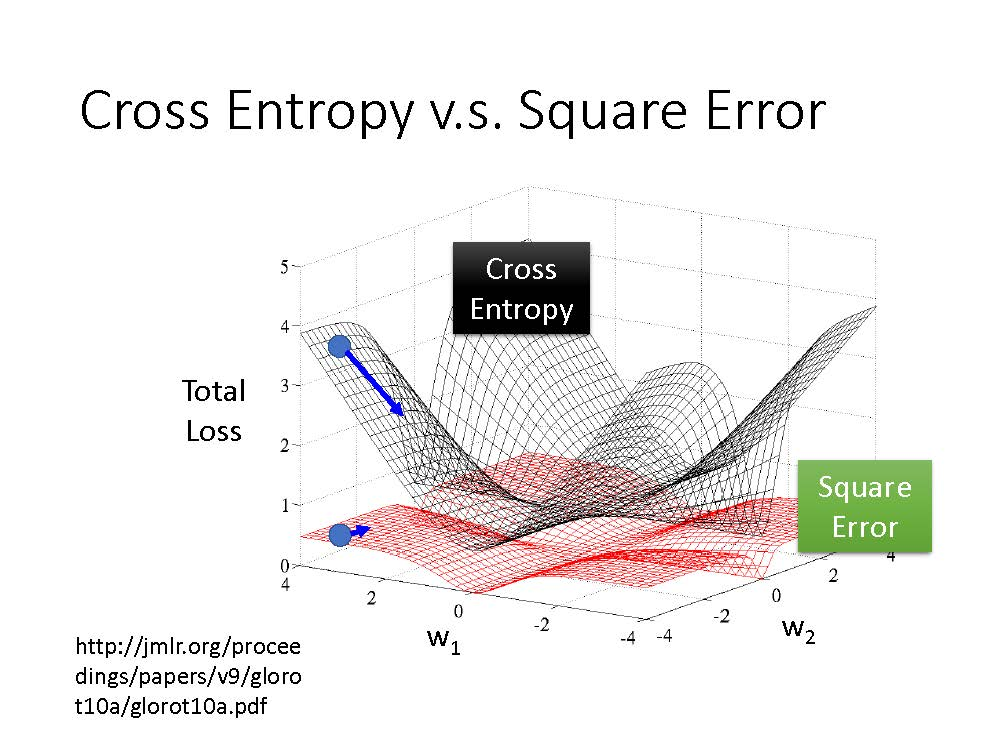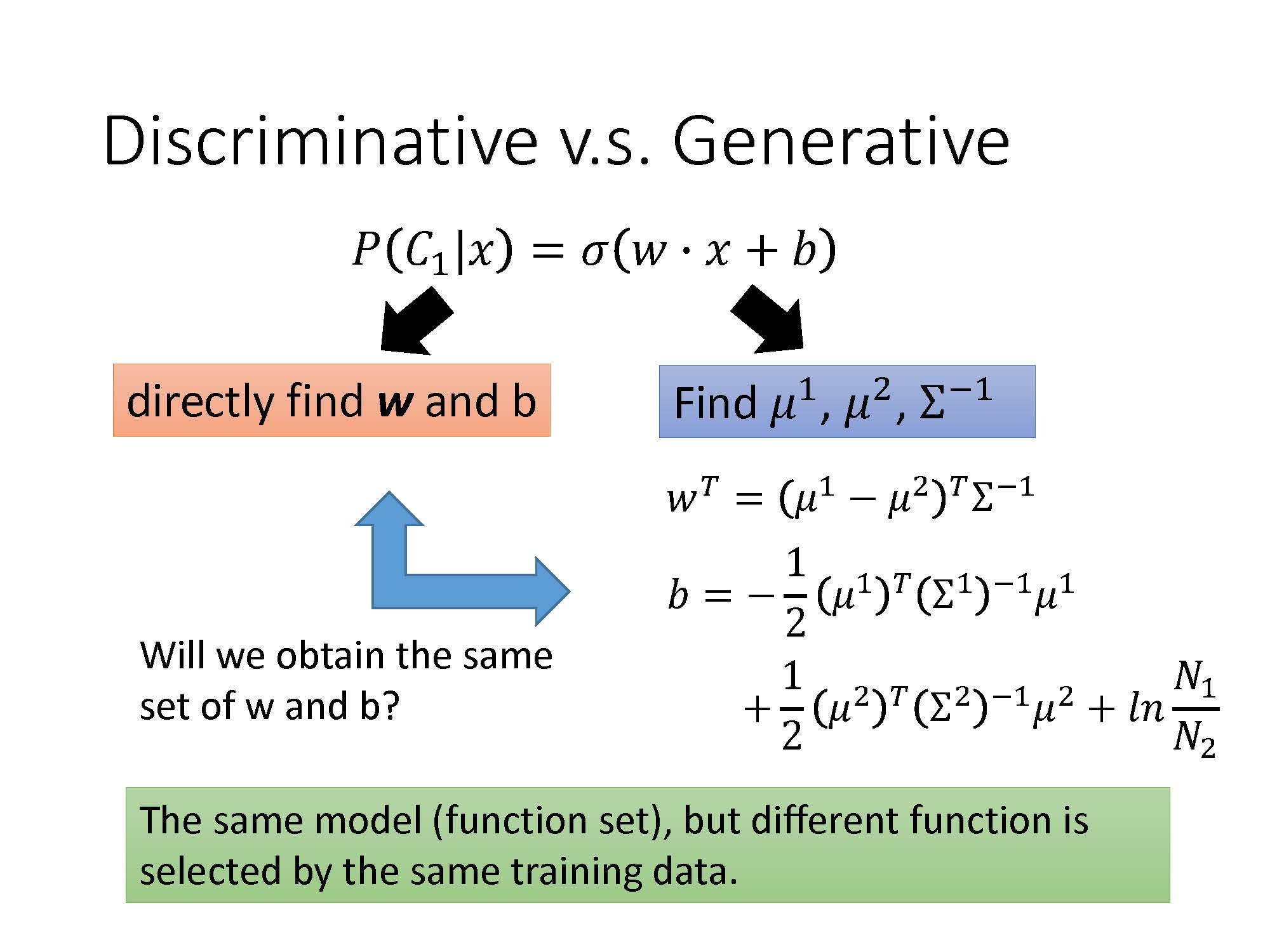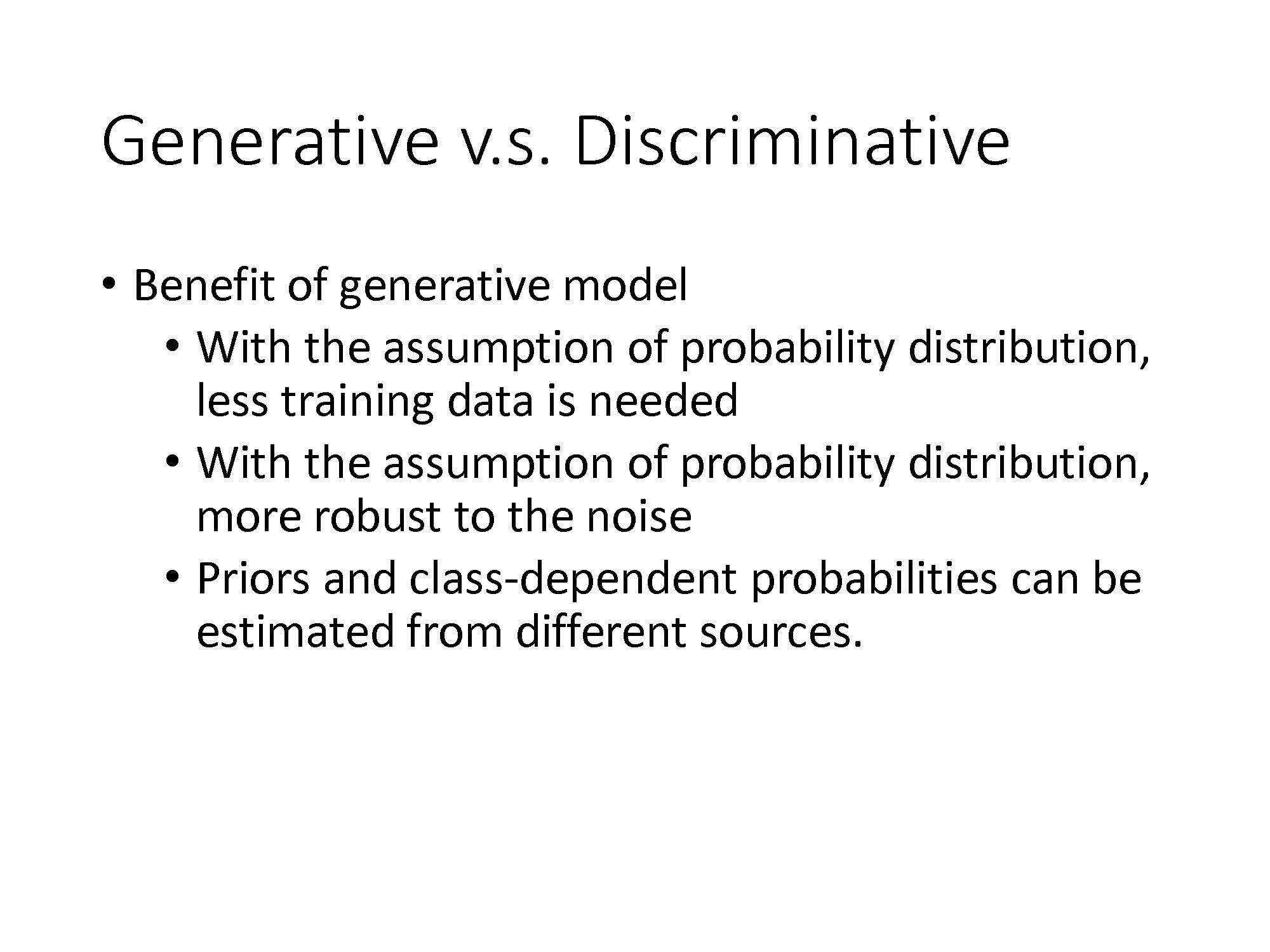softmax(zj)=ezjiezi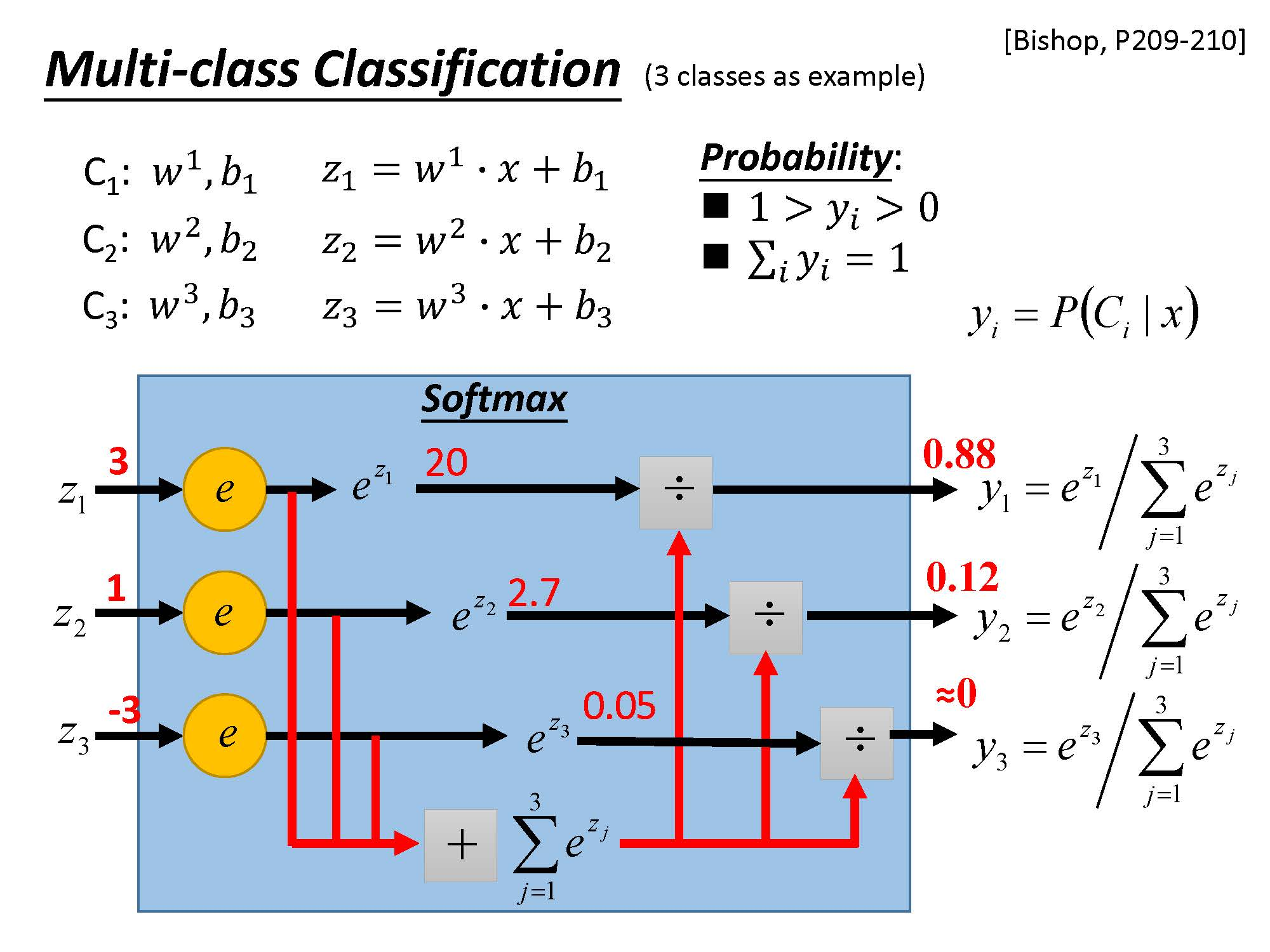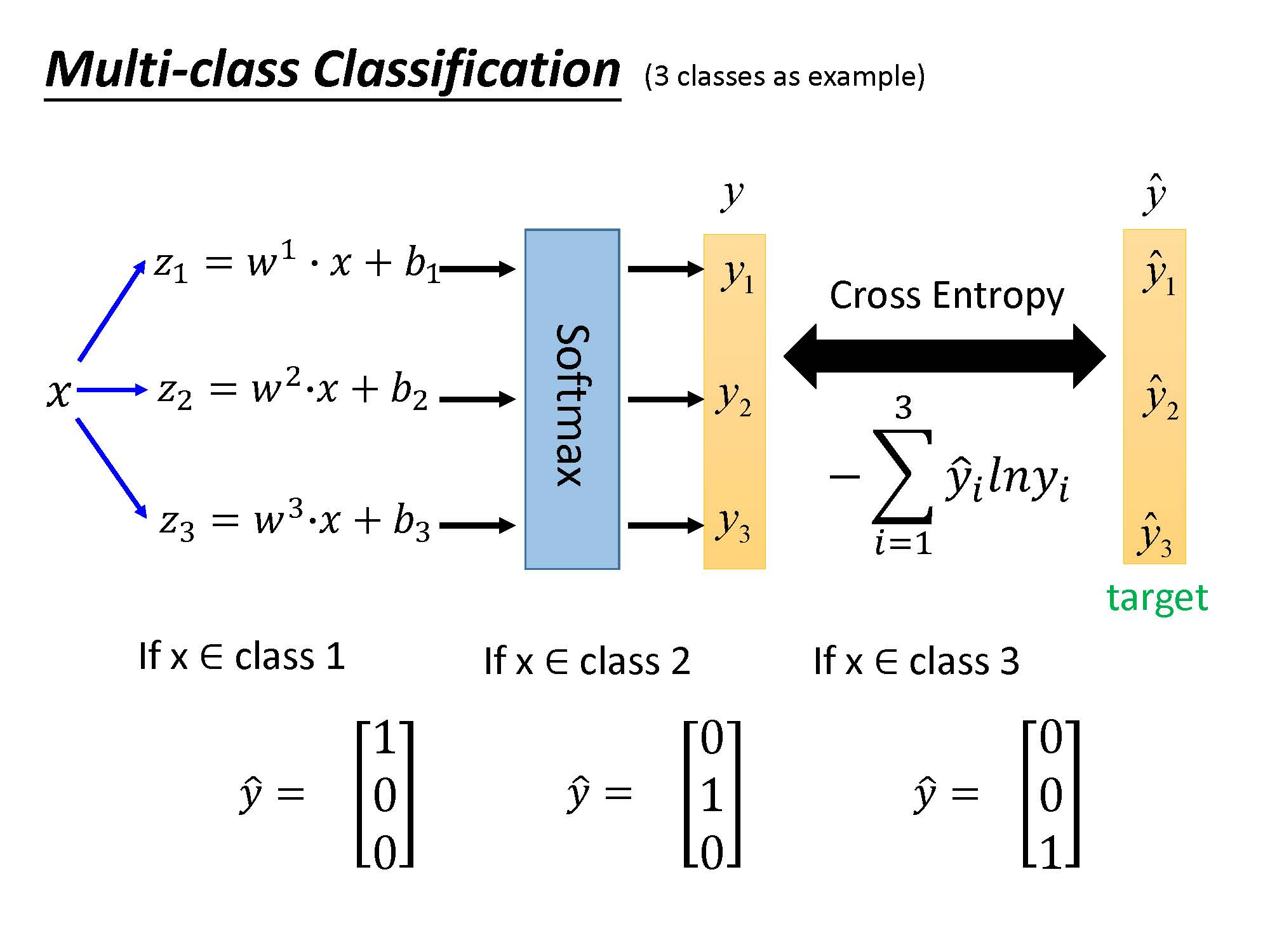Logistic Regression$Logistic\ Regression$的限制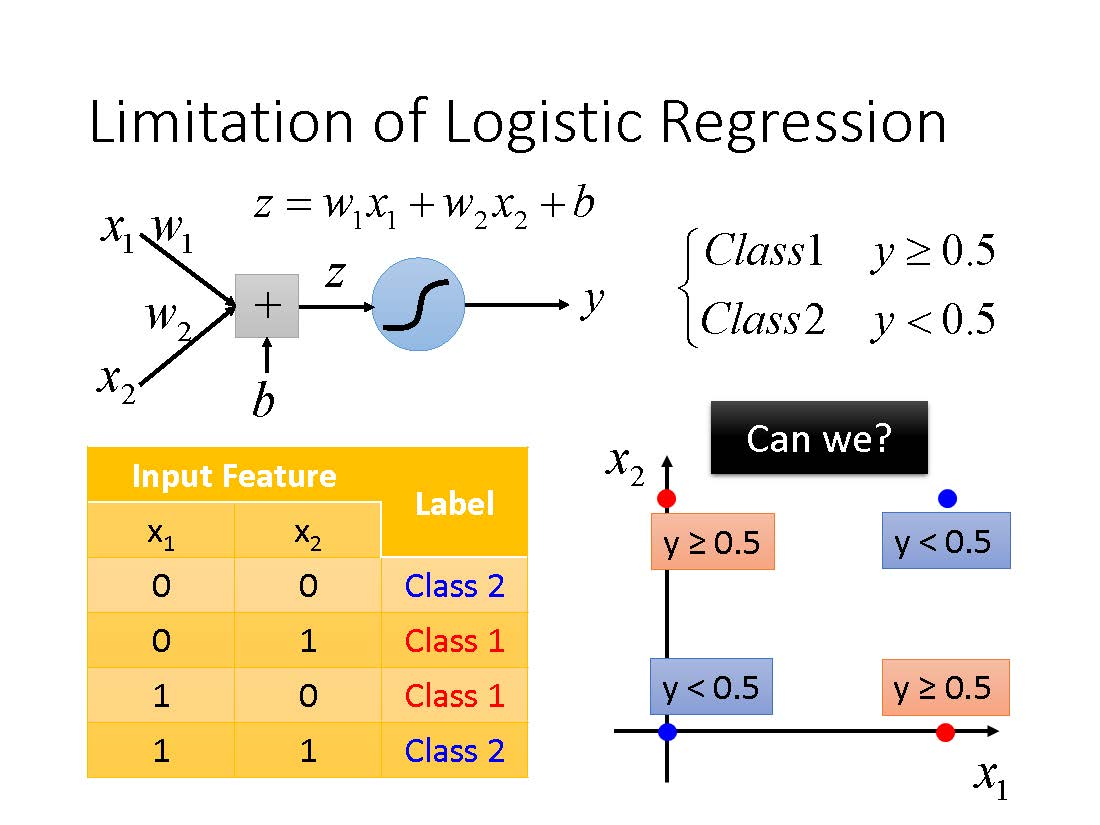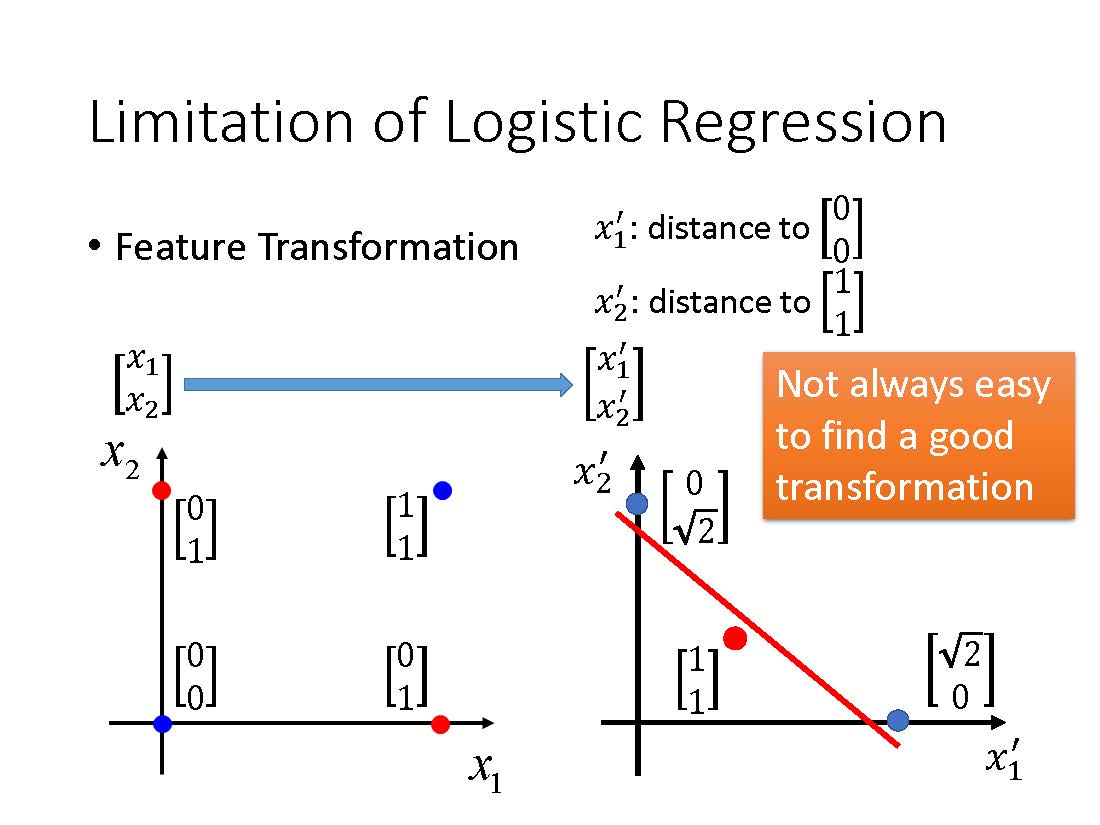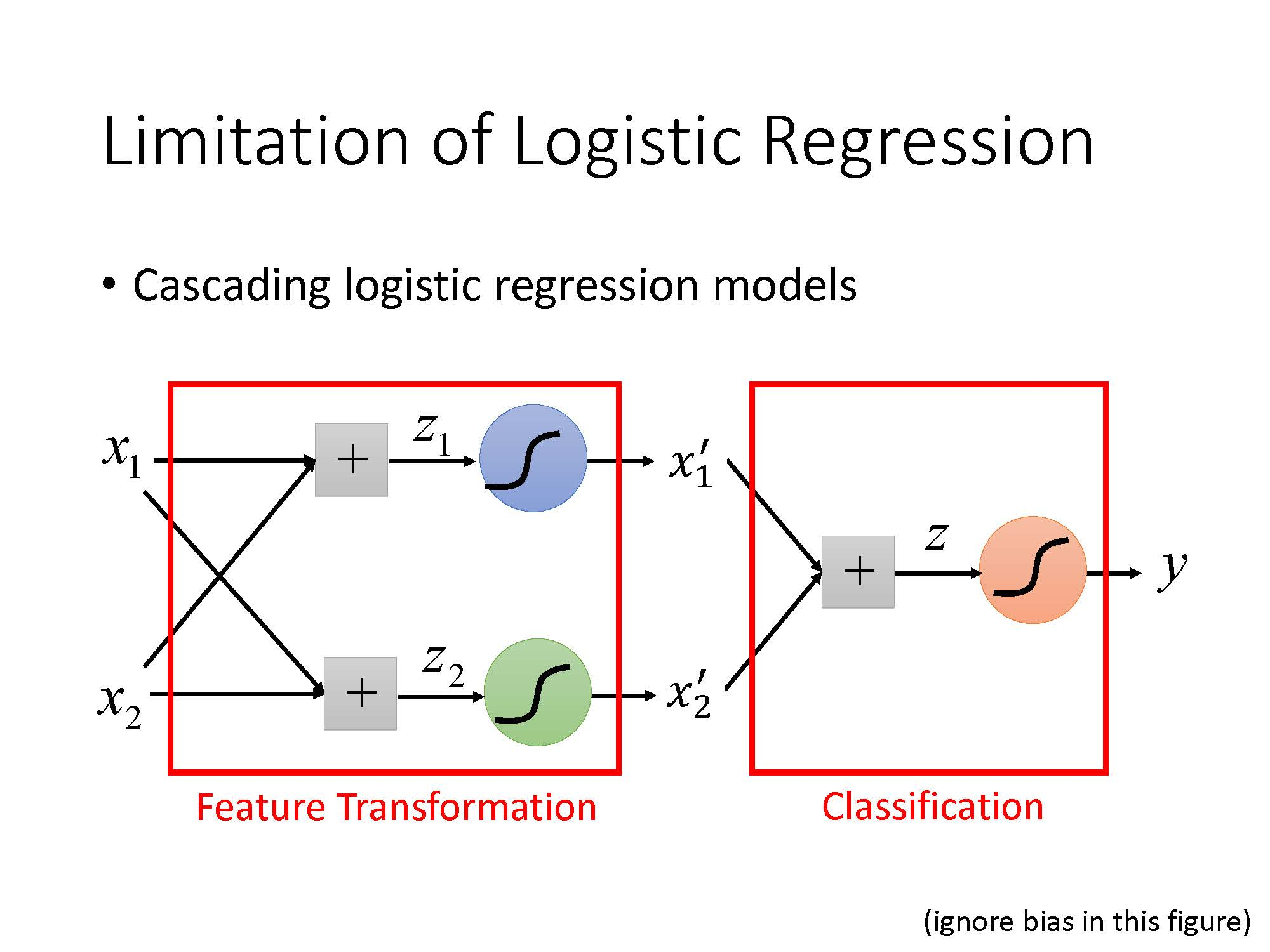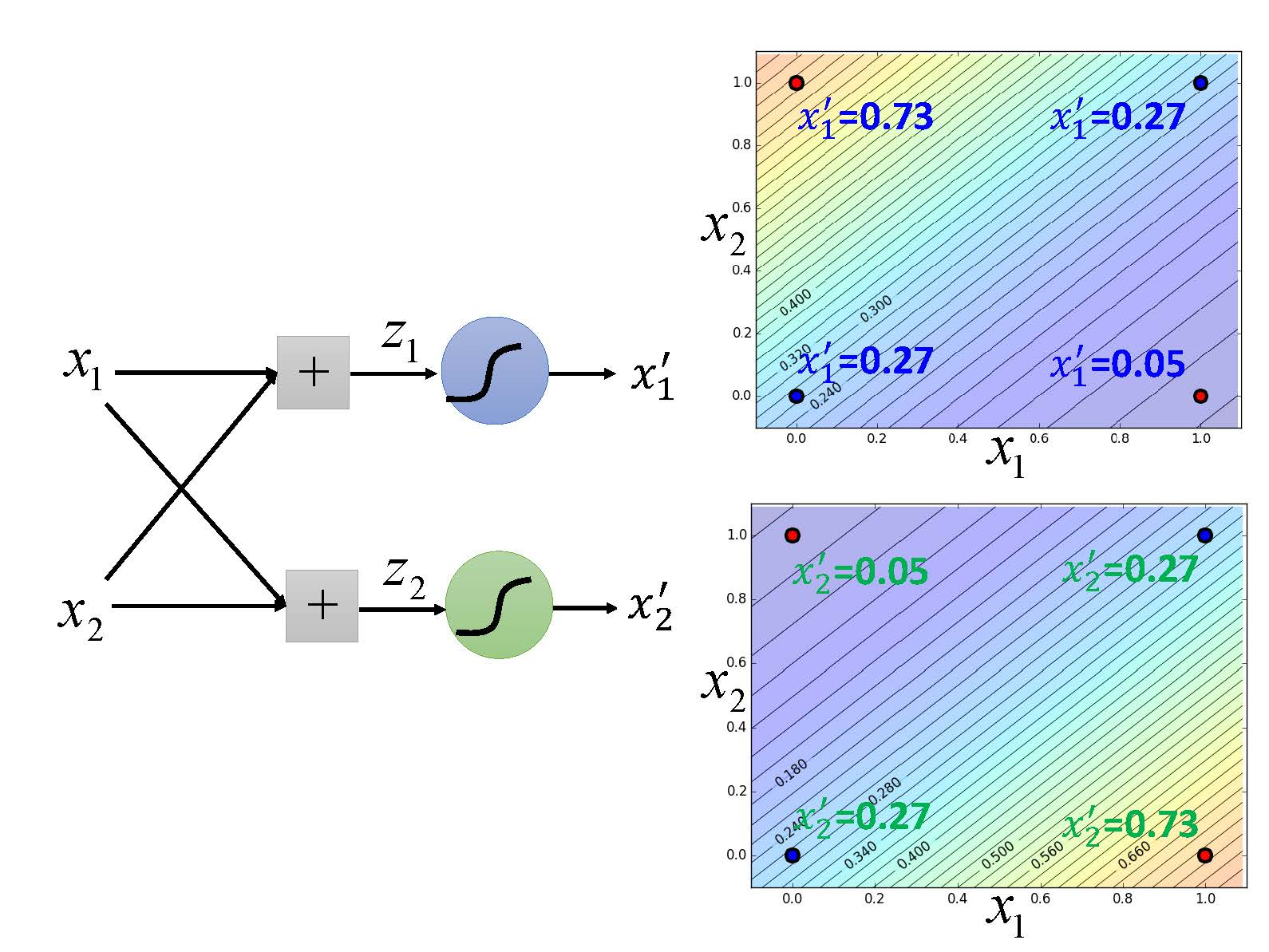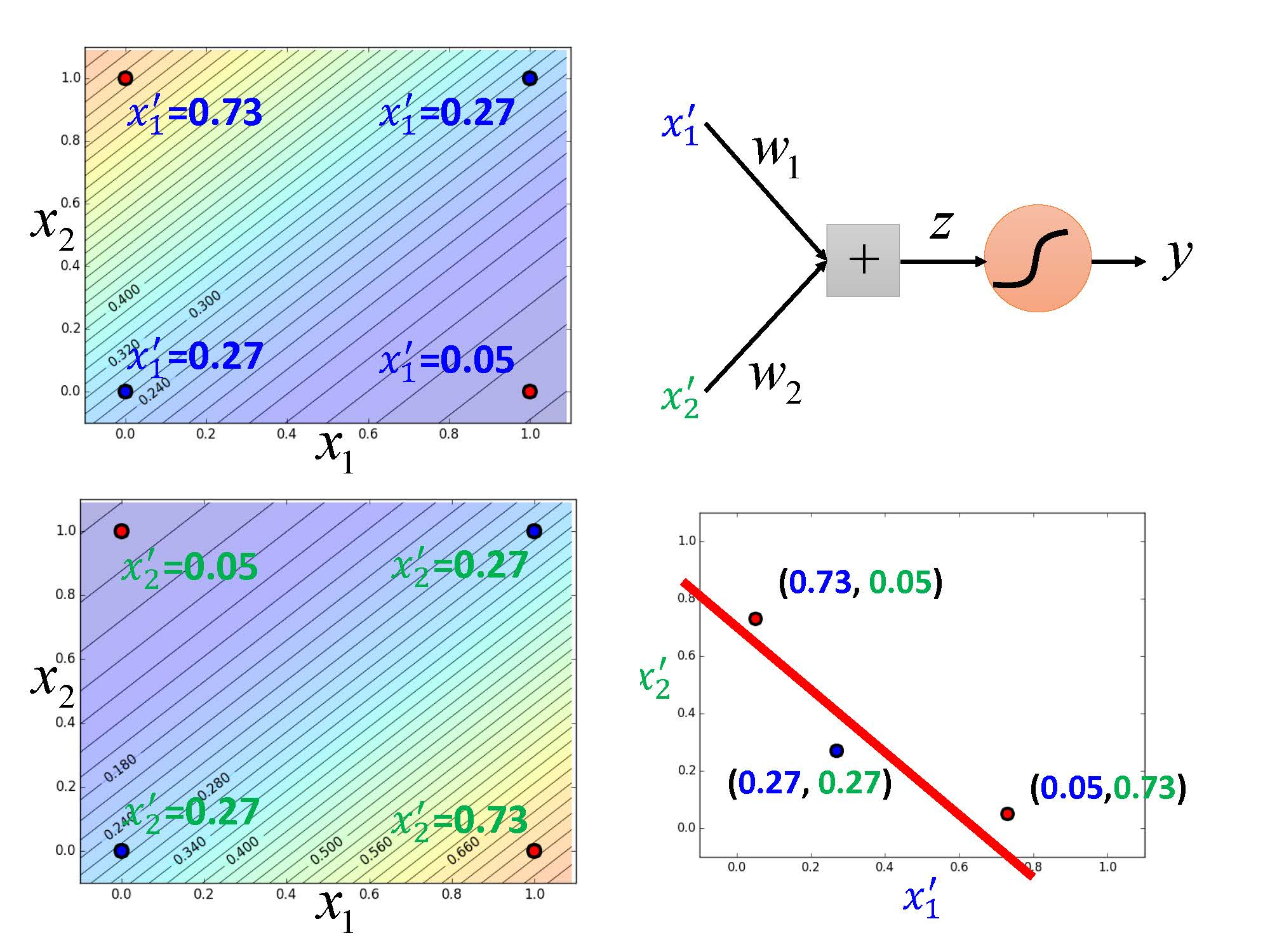©️2019 CSDN 皮肤主题: 编程工作室 设计师: CSDN官方博客